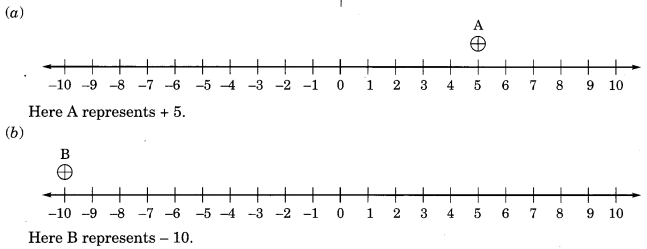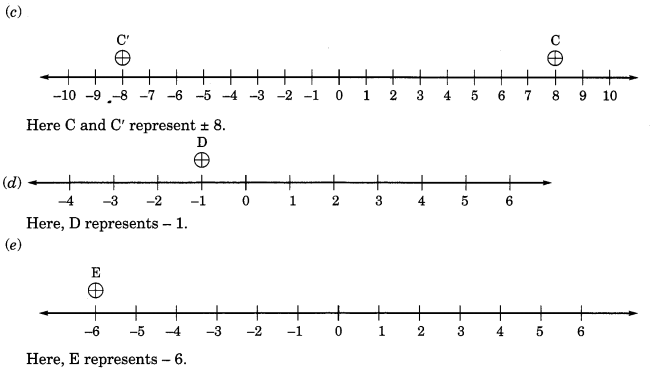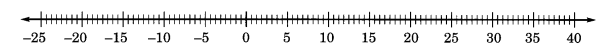# NCERT Solutions for Class 6 Maths Chapter 6 Integers Ex 6.1

ncert textbook

## NCERT Solutions for Class 6 Maths Chapter 6 Integers Ex 6.1

NCERT Solutions for Class 6 Maths Chapter 6 Integers Ex 6.1

Exercise 6.1

Question 1.
Write opposites of the following:
(a) Increase in weight
(b) 30 km North
(c) 326 BC
(d) Loss of ₹700
(e) 100 m above sea level.
Solution:
(a) Decrease in weight
(b) 30 km South
(d) Profit of ₹700
(e)100 m below sea level.

Question 2.
Represent the following numbers as integers with appropriate signs.
(a) An aeroplane is flying at a height two thousand metre above the ground.
(b) A submarine is moving at a depth, eight hundred metre below the sea level.
(c) A deposit of rupees two hundred.
(d) Withdrawal of rupees seven hundred.
Solution:
(a) +2000 m
(b) -800 m
(c) + ₹200
(d) – ₹700

Question 3.
Represent the following numbers on a number line: ,
(a) +5
(b) -10
(c) ±8
(d) -1
(e) -6
Solution:Question 4.
Adjacent figure is a vertical number line, representing integers. Observe it and locate the following points:(a) If point D is +8, then which point is -8?
(b) Is point G a negative integer or a positive integer?
(c) Write integers for points B and E.
(d) Which point marked on this number line has the least value?
(e) Arrange all the points in decreasing order of value.
Solution:
(a) F represents -8
(b) G is a negative integer.
(c) B represents +4 and E represents – 10
(d) E has the least value of -10.
(e) Decreasing order of all the points are: D, C, B, A, 0, H, G, F and E.

Question 5.
Following is the list of temperatures of five places in India on a particular day of the year.(a) Write the temperatures of these places in the form of integers in the blank column.
(b) Following is the number line representing the temperature in degree Celsius.Plot the name of the city against its temperature.
(c) Which is the coolest place?
(d) Write the names of the places where temperatures are above 10°C.
Solution:(c) Siachin is the coolest place with -10°C temperature.
(d) (i) Delhi → 20°C

Question 6.
In each of the following pairs, which number is to the right of the other on the number line?
(a) 2, 9
(b) -3, -8
(c) 0, -1
(d) -11, 10
(e) -6, 6
(f) 1, -100
Solution:
(a) 9 is to the right of 2
(b) -3 is to the right of -8
(c) 0 is to the right of – 1
(d) 10 is to the right of -11
(e) 6 is to the right of -6
(f) 1 is to the right of -100.

Question 7.
Write all the integers between the given pairs (write them in the increasing order):
(a) 0 and -7
(b) -4 and 4
(c) -8 and -15
(d) -30 and -23
Solution:
(a) Integers between 0 and -7 are:
– 6, – 5, – 4, -3,-2,- 1.
(b) Integers between -4 and 4 are:
– 3, – 2, -1, .0, 1, 2, 3.
(c) Integers between -8 and -15 are:
-14,-13, -12,-11,-10,-9.
(d) Integers between -30 and -23 are:
– 29, – 28, – 27, – 26, – 25, – 24.

Question 8.
(a) Write four negative integers greater than -20.
(b) Write four negative integers less than – 10.
Solution:
(a) Four negative integers greater than – 20 are: – 19, – 18, – 17, – 16.
(b) Four negative integers less than – 10 are: – 11, – 12, – 13, – 14.

Question 9.
For the following statements, write True (T) or False (F).
If the statement is false, correct the statement.
(a) – 8 is to the right of – 10 on a number line.
(b) – 100 is to the right of- 50 on a number line.
(c) Smallest negative integer is – 1
(d) – 26 is greater than – 25.
Solution:
(a) True (T)
(b) False (F); Correction: -100 is to the left of -50 on a number line.
(c) False (F); Correction: There is no smallest negative integer.
(d) False (F); Correction: -26 is smaller than -25.

Question 10.
Draw a number line and answer the following:
(a) Which number will we reach if we move 4 numbers to the right of -2.
(b) Which number will we reach if we move 5 numbers to the left of 1.
(c) If we are at -8 on the number line, in which direction should we move to reach -13?
(d) If we are at -6 on the number line, in which direction should we move to reach -1?
Solution: